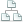Home >Tips >Excel 2016 from Scratch >Vertical lookup C – Function guideSite map

# Excel 2016 from Scratch Vertical Lookup C – Function Guide

## An Excel tutorial by Peter KalmstromIn this exercise in the Excel 2016 from Scratch series, Peter Kalmstrom shows more in detail how he used the Excel function guide to create a formula with a vertical lookup in the previous article.

This demo uses the same example exercise as the Vertical Lookup B article, but Peter does not go through all the necessary calculations done in the exercise. He just explains more in thoroughly how to use the function guide, in this case to create a formula that contains a vertical lookup.

Peter uses Excel 2016 for his demo, but the Excel calculation functions are the same for earlier versions of Excel.